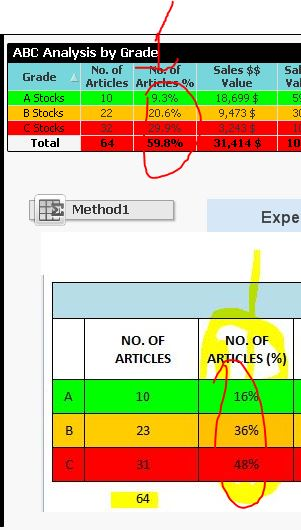# QlikView App Dev

Discussion Board for collaboration related to QlikView App Development.

Announcements
cancel
Showing results for
Did you mean:Master II

## Total Count % issue

Hi,

tried to applied below calculated dimension and it's not working for % column. is there anyway to resolve this issue? thanks

Requirement: if article Sold<=0 and soh<=0 then exclude else show the county by Grade (Calculated dimension)

Current Cal. Dimension:

=if(aggr(sum({\$<Year={"\$(=max(Year))"},Week={"\$(=max(Week))"}>}SoldQty),ArticleNo)<=0 or

aggr(sum({\$<Year={"\$(=max(Year))"},Week={"\$(=max(Week)+1)"},ShopNo-={'xxxxx'}>}soh),ArticleNo)<=0,Null(),

Aggr(If(rangesum(above(TOTAL (Sum({<ArticleNo>} {\$<Year={"\$(=Max(Year))"},Week={"\$(=Max(Week))"}>}TotalNetPriceSGD)/

Sum(TOTAL {<ArticleNo>} {\$<Year={"\$(=Max(Year))"},Week={"\$(=Max(Week))"}>} TotalNetPriceSGD)),0,RowNo(Total)))<=0.6,'A Stocks',

if(rangesum(above(TOTAL (Sum({<ArticleNo>} {\$<Year={"\$(=Max(Year))"},Week={"\$(=Max(Week))"}>}TotalNetPriceSGD)/

Sum(TOTAL {<ArticleNo>}{\$<Year={"\$(=Max(Year))"},Week={"\$(=Max(Week))"}>} TotalNetPriceSGD)),0,RowNo(Total)))>0.6

and rangesum(above(TOTAL (Sum({<ArticleNo>} {\$<Year={"\$(=Max(Year))"},Week={"\$(=Max(Week))"}>}TotalNetPriceSGD)/

Sum(TOTAL {<ArticleNo>} {\$<Year={"\$(=Max(Year))"},Week={"\$(=Max(Week))"}>} TotalNetPriceSGD)),0,RowNo(Total)))<=0.9,'B Stocks','C Stocks')),

(ArticleNo, (=Sum({<ArticleNo>} {\$<Year={"\$(=Max(Year))"},Week={"\$(=Max(Week))"}>}TotalNetPriceSGD), DESC)))

)

Measure:

No. of Article %

Count(DISTINCT ArticleNo)/ Count(total DISTINCT ArticleNo)

Note: above expression tried to merge with set analysis and count wrong and it's not working also (refer to the Method 1 chart object)

fyi, below 1st picture is the current o/p and bottom on is the expected output. thanks1 Solution

Accepted SolutionsMVP

Did you get to check my email?

Is this working

[No. of Articles]/Sum(TOTAL Aggr(If(Aggr(Sum({\$<Year={"\$(=max(Year))"},Week={"\$(=max(Week))"}>}SoldQty),ArticleNo)<=0 or

'CKS-WRTOFF','CKS-MKT','BRAND-MKT','CKS-CUST SVC','CKI-MKT','VSloc EC','VSloc CN','CKS-PS-DI','CK-MACAU'}>}soh),ArticleNo)<=0, Null(), Count(DISTINCT ArticleNo)), ArticleNo))MVP

Did you get to check my email?

Is this working

[No. of Articles]/Sum(TOTAL Aggr(If(Aggr(Sum({\$<Year={"\$(=max(Year))"},Week={"\$(=max(Week))"}>}SoldQty),ArticleNo)<=0 or

'CKS-WRTOFF','CKS-MKT','BRAND-MKT','CKS-CUST SVC','CKI-MKT','VSloc EC','VSloc CN','CKS-PS-DI','CK-MACAU'}>}soh),ArticleNo)<=0, Null(), Count(DISTINCT ArticleNo)), ArticleNo))